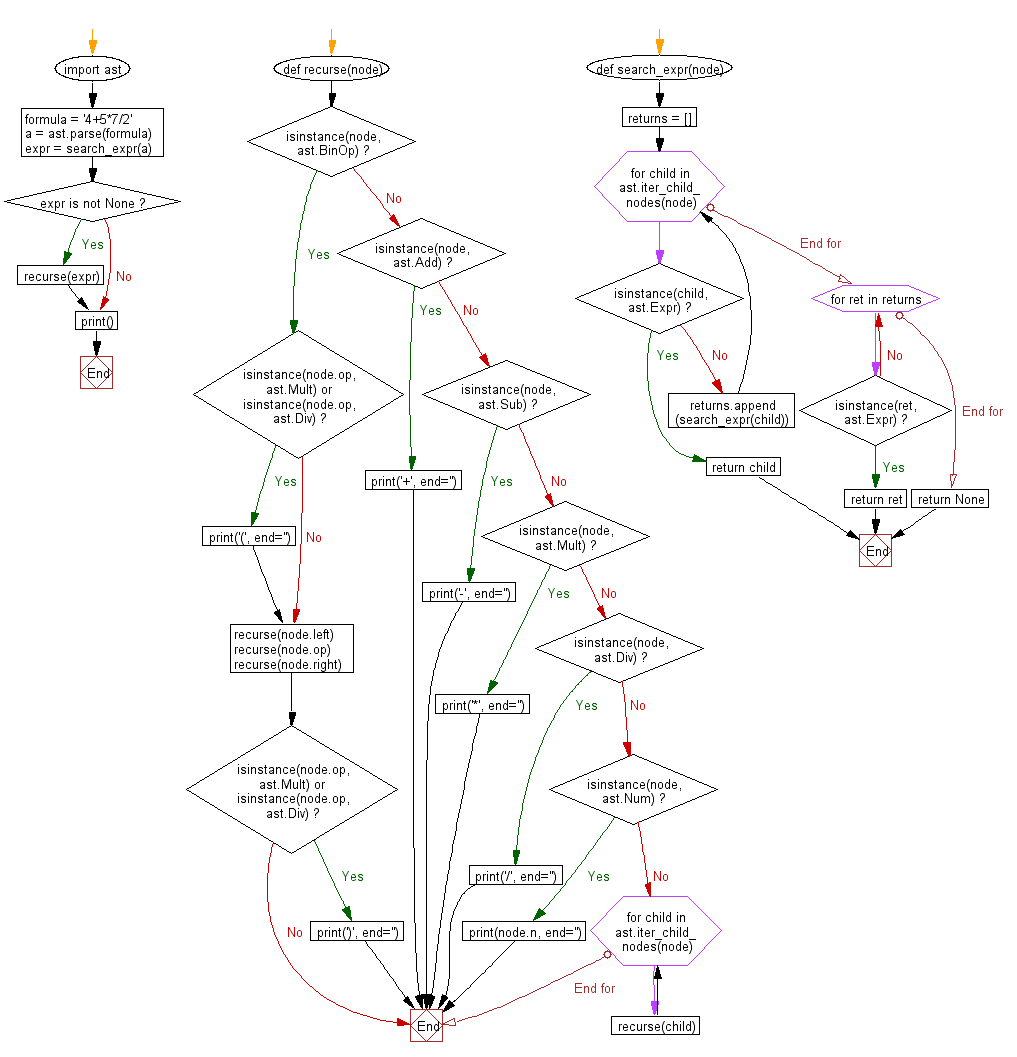﻿ Python Math: Parse math formulas and put parentheses around multiplication and division - w3resource# Python Math: Parse math formulas and put parentheses around multiplication and division

## Python Math: Exercise-60 with Solution

Write a Python program to parse math formulas and put parentheses around multiplication and division.

Sample Solution:-

Python Code:

``````#https://gist.github.com/ohaz/ed0b14a487b0569aad2d
import ast

def recurse(node):
if isinstance(node, ast.BinOp):
if isinstance(node.op, ast.Mult) or isinstance(node.op, ast.Div):
print('(', end='')
recurse(node.left)
recurse(node.op)
recurse(node.right)
if isinstance(node.op, ast.Mult) or isinstance(node.op, ast.Div):
print(')', end='')
print('+', end='')
elif isinstance(node, ast.Sub):
print('-', end='')
elif isinstance(node, ast.Mult):
print('*', end='')
elif isinstance(node, ast.Div):
print('/', end='')
elif isinstance(node, ast.Num):
print(node.n, end='')
else:
for child in ast.iter_child_nodes(node):
recurse(child)

def search_expr(node):
returns = []
for child in ast.iter_child_nodes(node):
if isinstance(child, ast.Expr):
return child
returns.append(search_expr(child))
for ret in returns:
if isinstance(ret, ast.Expr):
return ret
return None

formula = '4+5*7/2'

a = ast.parse(formula)

expr = search_expr(a)
if expr is not None:
recurse(expr)
print()
```
```

Sample Output:

```4+((5*7)/2)
```

Flowchart:Python Code Editor:

Have another way to solve this solution? Contribute your code (and comments) through Disqus.

What is the difficulty level of this exercise?

Test your Programming skills with w3resource's quiz.

﻿

```>>> students = [{'name': 'John', 'score': 98}, {'name': 'Mike', 'score': 94}, {'name': 'Jennifer', 'score': 99}]